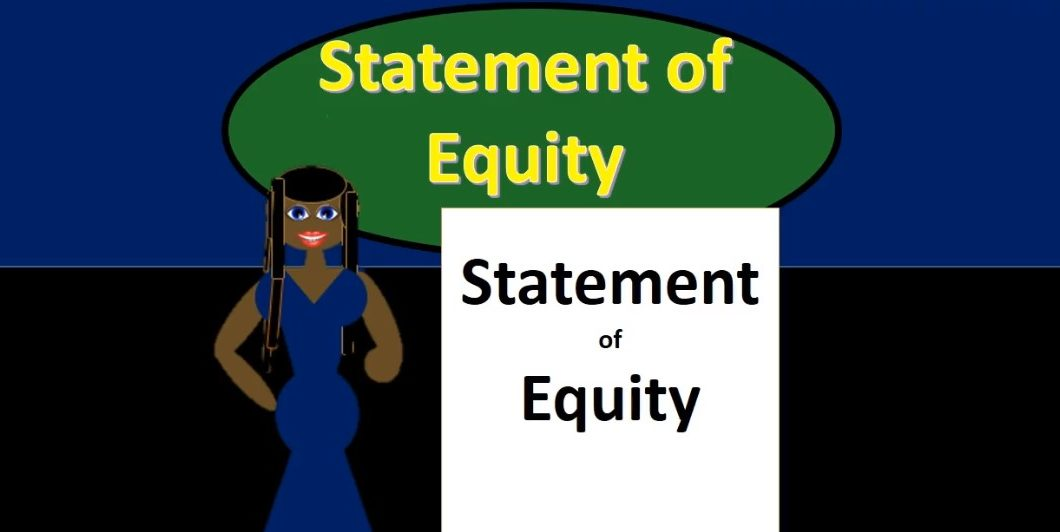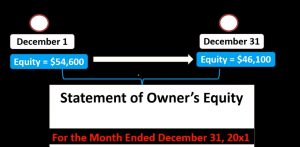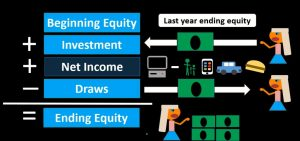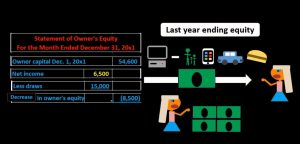## Statement of Owner’s Equity 131

Hello in this presentation we will describe the statement of equity objectives, we will be able to at the end of this describe the statement of owner’s equity, list the components of the statement of owner’s equity and explain the reasons for a statement of owner’s equity. When we consider the statement of owner’s equity, we are like the income statement and unlike the balance sheet, talking about a timeframe, meaning we have a beginning and end point, unlike the income statement, the beginning point is not zero meaning we are going to start at the beginning point of the net value or the equity section of the prior balance.

00:42

So what we are looking at is where was the company on an equity basis where was the company in terms of net assets in terms of raw assets minus liabilities as of the beginning of the time period, so in this case, December 31, and then what happened in turn Have the activity during that time period. And then where did we leave off at the end of the time period. So note that we have two distinct points here in time that we are looking into one is a distinct point where we’re showing the value of the company in terms of book value basis assets minus liabilities, at the beginning of the time period, and the value of the company on a book basis as of the end of the time period. And of course, we’re going to reconcile the difference between those two by showing the activity during this time period. So that is going to be done with a statement of owner’s equity.01:40

And we’re going to have the same kind of phrasing we would have on the income statement. So of course we’re going to have for the month ended, we cannot just have the date of December 31 2001. In this case, as we would have on the balance sheet, we need for the month ended. We need that beginning timeframe and that ending timeframe, that beginning point in time. And that ending point in time, note that in this case, the equity actually went down. So we have beginning equity of 54 600. And we ended at 46 100. So we need to basically see what happened in the interim in terms of decreasing the total equity. So let’s take a look at the calculation.

02:21

The calculation will be beginning equity, where we started at last year’s ending equity, then we’re going to have investments, so any investments from the owner will increase this calculation. If we move to corporations, of course, we’re talking about the selling of stock. In essence, the calculation is going to be much the same. When we look at different types of entities in terms of a sole proprietor, a partnership, we’re going to have just multiple partners, but the essence of the calculations in terms of equity as a total will be the same. And the names of a corporation in terms of how does an owner invest in a company when we are a corporation? And how to draw is how In terms of dividends in a corporation will be a difference in terms of names, then we’re going to have an increase in net income.

03:08

So net income is going to increase the activity, that’s going to be the main factor typically. And then we’re going to in this case, we had service income, which is going to be services minus the expenses, meaning we had revenue from service revenue, minus the expenses, this entire amount being reflected on the income statement. So we’re not actually going to show the detail here of the revenue minus expenses that is shown on the income statement. What we will show as a reconciling item of the beginning balance to the ending balance is that net income that then can be expanded and explored more when going into an income statement. Then we’re going to decrease this amount by draws, draws representing the amount that the owner has drawn out of the company taken out for personal use.

03:56

In a corporation it would be similar to dividends, dividends, being the amount of money being distributed to the owners, to the shareholders for their personal use. And that would then give us our ending balance. So then we have our ending balance. And we can think of it as what is owed or contributed to the owner, meaning kind of the book value of the company, you can think of it as if we were to sell all the assets and get the cash and then pay off all the liabilities, that would then be what would be left over, if we had sold all the assets for exactly what the assets value were for, and pay off all the liabilities for the value that they were, that would be the Indian book value. So our calculation once again is always going to be for the equity section.

04:43

We’re going to start off at beginning equity. Like it’s like the income statement, but we’re not starting at zero. We’re starting at the Indian point of where we were last time, last times Indian equity, this times beginning equity, and then we’re going to reconcile the activity that happened in order to get us to the ending point the Indian equity, the number that then is going to be reported on the balance sheet. Therefore, we’re going to take beginning of equity, we’re going to increase the amount that the owner put in to their business, that’s going to increase the amount of equity increase the amount that’s owed back to the owner, we’re going to increase it by net income, revenue minus expenses, the earnings of the company, this should be the biggest factor typically, because this is what happened during a particular time period, usually a month or a year, then we’re going to subtract out any draws that happened in terms of money going out to the owner, if the money went out to the owner, then the total equity that is representing the amount due to the owner or assets minus liabilities has been decreased.05:46

That will then give us our Indian equity balance. So if we look at a statement of equity, a very kind of simple statement of equity we start with the beginning balance in this case, note the dates were have for the month ended December 31. That of course means That the month is beginning at December 1 and ending at December 31. We’re going to take the last year’s Indian equity, that’s going to be this number, starting with the owner capital account and taking the 54 600. And it’s going to be last year’s equity. Where would we get that number you might ask, we could look at the last year or in this case, we have the last year, but this is for a month. So this would be the last month. In our case, it would be November, we could be reporting, of course the statement of equity for a year’s time period or a month time period, this will be for a month time period.

06:35

So we could go back and look at November and say Hey, where did November end. And that should be our beginning point. But typically we will look at our trial balance and the trial balance. Oftentimes, the amount reported in the capital account is the beginning capital. And it’s either just the beginning capital or it will include investments and we will have to go to the to the General Ledger and see if there’s any activity during the time period. If there’s no activity during the time period, then the amount reported on the trial balance will be basically the beginning balance, even though the trial balance typically does not report the date on the trial balance. So just keep that in mind. When you’re putting these things together, you typically will be putting them together from a trial balance taking this number directly from the trial balance, even though there’s no date next to it on the trial balance and adding, you know, December 1 to it, then in this case, we’re going to increase it by net income.

07:33

So net income being a number that we are going to get from the income statement. This number here should be the bottom line, last number on the income statement, and then we’re going to decrease it by draws. So note here that the draws are actually much larger than net income. So although net income is increasing the 6500 net income minus the 15,000 draw It actually results in that decrease in owner’s equity. So we have a decrease in owner’s equity because more was drawn out of the business than was earned in net income for this time period, meaning earnings were 6500, the owner drew out for personal use 15,000, we might think that that would be a very big problem. And if it was the first year of operations, it could be a very big problem.

08:25

But in this case, note that we had retained a nurse prior to this of 54,600. So even though we pulled out a lot more 15,000 then earned 6500 there’s still a lot of value within the company, meaning we still have the 54,600 beginning balance minus the net here of 8500, leaving us with a net balance of 46,100. So there’s still value in in the company here at even though more was pulled out than was earned in this current month. This 46,100 would then be what is reported on the balance sheet. Also note that this does represent this 46,100 represents the book value of the company. And you if that would lead us to think that the owner could at any time pull out 46,100.09:19

But note that that’s not necessarily the case typically not because the organization does not have the business does not have \$46,100 in cash, what they do have is 46,100 of net assets, meaning we have total assets measured in dollars, including cash, but also including equipment and other things that are non cash minus liabilities of 46,100. So, note that although we have this much that we can say the owner has claimed to the owner would not be able to take that much money out of the organization Unless Unless they had basically We liquidated the organization in terms of selling the assets, receiving the cash and then paying off the liabilities. So just keep in mind that this is the bottom line number, the the kind of the, the main number on the balance sheet and on the statement of equity representing the where a company stands as of a point in time, but that does not mean that the owner can walk away with that at any given point in time knowing that that of course, is including assets minus liabilities, all assets, not just not just the cash assets. objectives, we are now able to describe the statement of owner’s equity. list the components of the statement of owner’s equity and explain the reasons for a statement of owner’s equity.